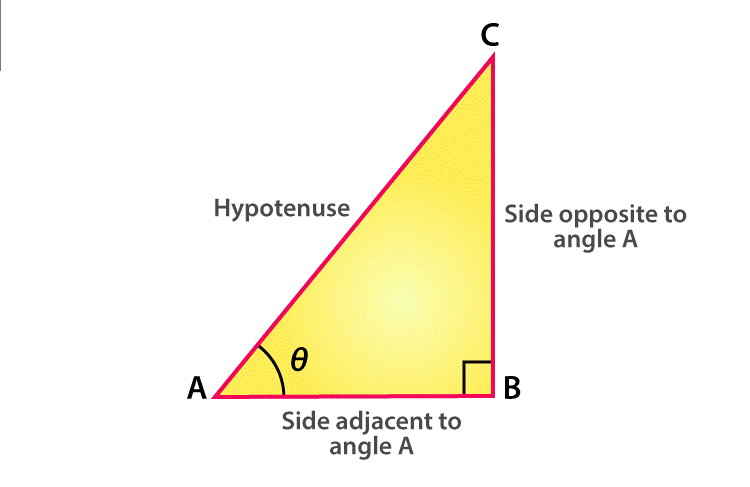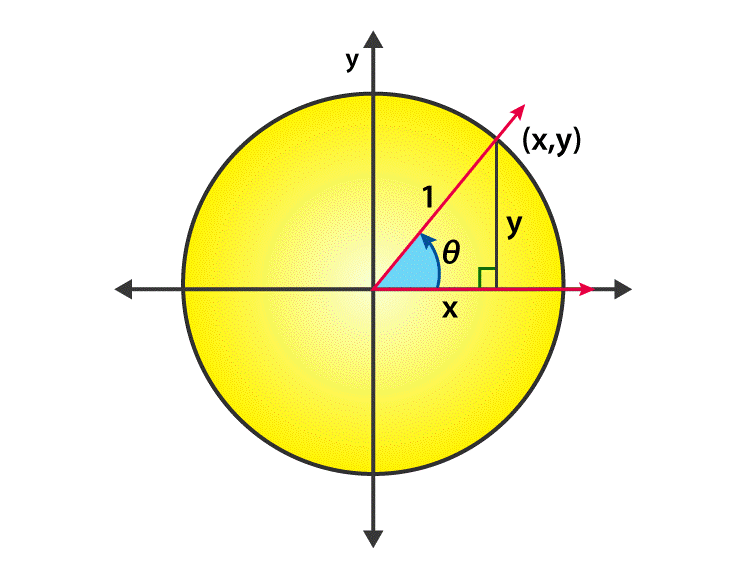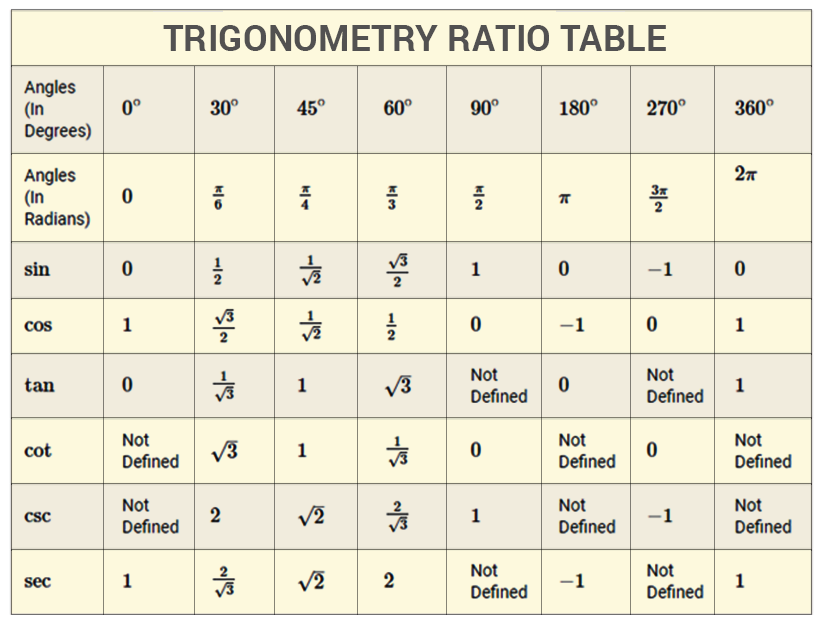# Sin 90 degrees

The trigonometric functions relates the angles of a triangle to the length of its sides. Trigonometric functions are most important in the study of periodic phenomena like sound and light waves and many other applications. The most familiar three trigonometric ratios are sine function, cosine function and tangent function. For angles less than a right angle, trigonometric functions are commonly defined as the ratio of two sides of a right triangle containing the angle and their values can be found in the length of various line segments around a unit circle.

The angles are calculated with respect to sin, cos and tan functions which are the primary functions whereas cosecant, secant and cot functions are derived from the primary functions. Usually, the degrees are considered as 00, 300, 450, 600, 900, 1800, 2700 and 3600. Here, we will discuss about the value for sin 90 degrees and how the values are derived along with other degrees or radian values.

## Sine 90 degrees value

To define the sine function of an acute angle, start with the right angled triangle ABC with the angle of interest and the sides of a triangle. The three sides of the triangle are given as follows:• The opposite side – side opposite to the angle of interest.
• The hypotenuse side – opposite side of the right angle and it is always the longest side of a right triangle
• The adjacent side – remaining side of a triangle and it forms a side of both the angle of interest and the right angle

The sine function of an angle is equal to the length of the opposite side divided by the length of the hypotenuse side and the formula is given by

$\sin \theta =\frac{opposite side}{hypotenuse side}$

The sine law states that the sides of a triangle are proportional to the sine of the opposite angles.

$\frac{a}{\sin A}=\frac{b}{\sin B}=\frac{c}{\sin C}$

In the following cases, sine rule is used. Those conditions are

Case 1 : Given two angles and one side (AAS and ASA)

Case 2 : Given two sides and non included angle (SSA)

## Derivation to Find the Value of Sin 90 Degrees

Let us now calculate the value of sin 900. Consider the unit circle.That is the circle with radius 1 unit and it is center placed in origin.From the basic knowledge of trigonometry, we conclude that for the given right angled triangle with base measuring ‘x’ units and the perpendicular measuring ‘y’ units.

We know that ,

For any right angled triangle measuring with any of the angles, sine functions equals to the ratio of the length of the opposite side to the length of the hypotenuse side. So, from the figure

$\sin \theta$ = y/1

Start measuring the angles from the first quadrant and end up with 900 when it reaches positive y axis. Now the value of y becomes 1 since it touches the circumference of the circle. Therefore the value of y becomes 1.

$\sin \theta$ = y/1 = 1/1

Therefore Sin 90 degree equals to the fractional value of 1/ 1.

Sin 900 = 1

The most common trigonometric sine functions are

• Sin 90 degree plus theta
$\sin (90^{\circ}+\theta )=\cos \theta$
• Sin 90 degree minus theta
$\sin (90^{\circ}-\theta )=\cos \theta$

Some other trigonometric sine identities are as follows:

• $\sin x=\frac{1}{\csc x}$
• $\sin^{2}x+\cos ^{2}x=1$
• $\sin (-x)=-\sin x$
• Sin 2x = 2 sin x cos x

In the same way, we can derive other values of sin degrees like 00,300,450,600,900,1800,2700 and 3600. Below is the trigonometry table, which defines all the values of sine along with other trigonometric ratios.Sample Example

### Question 1:

Find the value of sin 1350

### Solution:

Given, sin 1350 = sin ( 900 + 450 )

= cos 450 (Since $\sin(90^{\circ}+\theta )$ =$\cos \theta$

= 1 /√2

Therefore, the value of sin 1350 is 1 /√2

### Question 2:

Find the value of cos 300 .

### Solution:

Given , cos 300 = sin ( 900 – 600 )

= Sin 600 (Since $\cos(90^{\circ}-\theta )$ =$\sin \theta$

= $\frac{\sqrt{3}}{2}$

Therefore, the value of cos 300 is $\frac{\sqrt{3}}{2}$.

Keep visiting BYJU’S for more information on trigonometric ratios and its related articles, and also watch the videos to clarify the doubts.